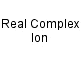Chromium(III) hexahydroxy ion
[Cr(OH)6]3-
Hexahydroxychromium(III) ionCr 1 H 6 O 6 Charge = -3

Real ion.

Gram formula weight (molecular mass) = 154.04

Hexahydroxychromium(III) ion is green.Hexahydroxychromium(III) ion
is defined with respect to the entities below:
The entities below are defined with respect to:
Hexahydroxychromium(III) ion
 Complex (AS/A2 generic)Real complex ion (generic)Type 23 Lewis acid/base complex (generic)VSEPR geometry = AX6 (generic)Weak acid (AS/A2 generic)chemical compound molecule metal molecular science reaction mechanism ionic material acid base geometry reactivity synthesis science knowledge chemistry Lewis